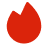# Indeed数据分析面试真题

BQ

Coding（2）
SQL（0）
Stats（0）
ML basics（0）
Product Case（0）高频题（0）

Coding（2）
SQL（0）
Stats（0）
ML basics（0）
Product Case（0）高频题（0）
1.LeetCode 1143
2.LeetCode 14
1. LeetCode 1143
Given two strings text1 and text2, return the length of their longest common subsequence. If there is no common subsequence, return 0.

A subsequence of a string is a new string generated from the original string with some characters (can be none) deleted without changing the relative order of the remaining characters.

• For example, "ace" is a subsequence of "abcde".
A common subsequence of two strings is a subsequence that is common to both strings.

Example 1:

```Input: text1 = "abcde", text2 = "ace"
Output: 3
Explanation: The longest common subsequence is "ace" and its length is 3.
```
Example 2:

```Input: text1 = "abc", text2 = "abc"
Output: 3
Explanation: The longest common subsequence is "abc" and its length is 3.
```
Example 3:

```Input: text1 = "abc", text2 = "def"
Output: 0
Explanation: There is no such common subsequence, so the result is 0.
```

Constraints:

• 1 <= text1.length, text2.length <= 1000
• text1 and text2 consist of only lowercase English characters.
2. LeetCode 14
Write a function to find the longest common prefix string amongst an array of strings.

If there is no common prefix, return an empty string "".

Example 1:

```Input: strs = ["flower","flow","flight"]
Output: "fl"
```
Example 2:

```Input: strs = ["dog","racecar","car"]
Output: ""
Explanation: There is no common prefix among the input strings.
```

Constraints:

• 1 <= strs.length <= 200
• 0 <= strs[i].length <= 200
• strs[i] consists of only lowercase English letters.